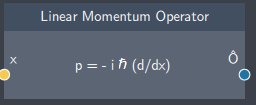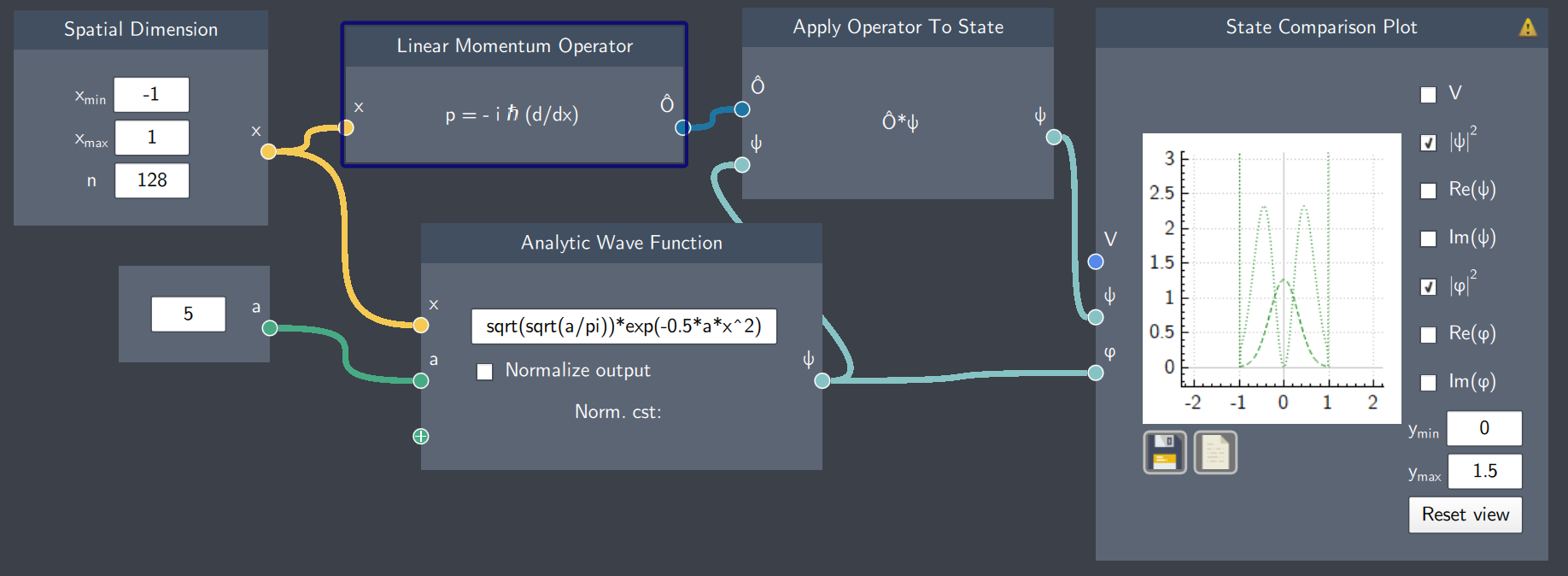# Linear Momentum Operator

## Description

The Linear Momentum Operator is the quantum mechanical operator which gives an instruction to differentiate with respect to $x$ (position) and multiply by $-i\hbar$.## Input

It has the following input:

• Spatial dimension ($x$): It inputs the values of $x$ defined in the Spatial Dimension node.

## Content

It consists of differentiated values of $x$ multiplied by $-i\hbar$.

## Output

• Linear Momentum Operator: The resulting linear momentum operator $\hat{p}$ which can be applied to a state.

## Example

In the example below, the Linear Momentum Operator node inputs the values of $x$and applies the operation to a Gaussian function which results in a new function. The magnitudes of both the Gaussian and the resulting function after operator application are shown on the plot.